Postulates Of Quantum Mechanics MCQ Level - 2

# Postulates Of Quantum Mechanics MCQ Level - 2

Test Description

## 10 Questions MCQ Test | Postulates Of Quantum Mechanics MCQ Level - 2

Postulates Of Quantum Mechanics MCQ Level - 2 for IIT JAM 2023 is part of IIT JAM preparation. The Postulates Of Quantum Mechanics MCQ Level - 2 questions and answers have been prepared according to the IIT JAM exam syllabus.The Postulates Of Quantum Mechanics MCQ Level - 2 MCQs are made for IIT JAM 2023 Exam. Find important definitions, questions, notes, meanings, examples, exercises, MCQs and online tests for Postulates Of Quantum Mechanics MCQ Level - 2 below.
Solutions of Postulates Of Quantum Mechanics MCQ Level - 2 questions in English are available as part of our course for IIT JAM & Postulates Of Quantum Mechanics MCQ Level - 2 solutions in Hindi for IIT JAM course. Download more important topics, notes, lectures and mock test series for IIT JAM Exam by signing up for free. Attempt Postulates Of Quantum Mechanics MCQ Level - 2 | 10 questions in 45 minutes | Mock test for IIT JAM preparation | Free important questions MCQ to study for IIT JAM Exam | Download free PDF with solutions
 1 Crore+ students have signed up on EduRev. Have you?
Postulates Of Quantum Mechanics MCQ Level - 2 - Question 1

### Consider a set of wave functions ψi(x). Which of the following condition guarantees that the functions are normalized and mutually orthogonal? (i, j  take values from 1 to n)

Detailed Solution for Postulates Of Quantum Mechanics MCQ Level - 2 - Question 1

For the states to be orthogonal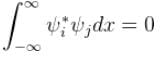and for them to be normalised,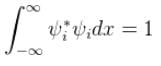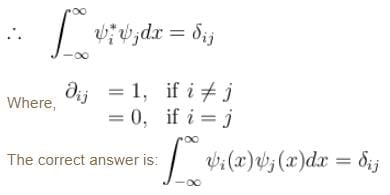Postulates Of Quantum Mechanics MCQ Level - 2 - Question 2

### The phase velocity of ripples on a liquid surface is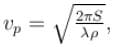where S is the surface tension and ρ the density of the liquid. The group velocity of the ripples is given by :

Detailed Solution for Postulates Of Quantum Mechanics MCQ Level - 2 - Question 2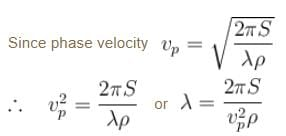As wave number,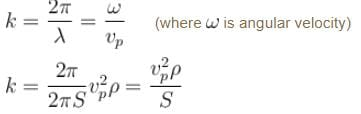We know that group velocity given by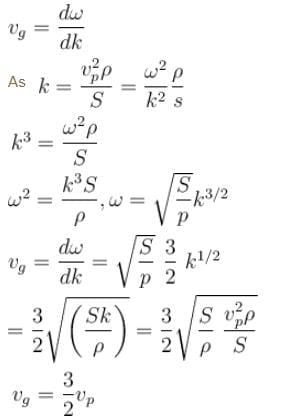The correct answer is: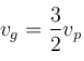Postulates Of Quantum Mechanics MCQ Level - 2 - Question 3

### The solutions of a free particle are :

Detailed Solution for Postulates Of Quantum Mechanics MCQ Level - 2 - Question 3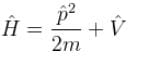V = 0 for a free particle
∴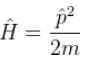Schrödinger equation.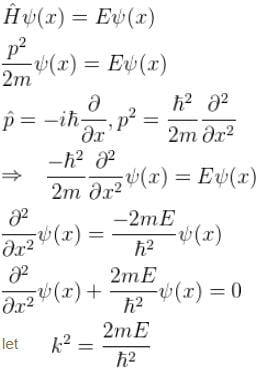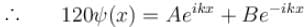(General solution)
∴    Aeikx   and  Be–ikx  are solution to the free particle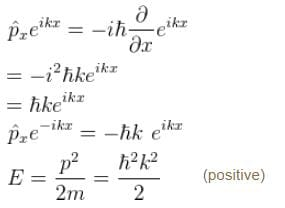The correct answer is: Energy and momentum eigen functions with both positive and negative momentum eigenvalues.

Postulates Of Quantum Mechanics MCQ Level - 2 - Question 4

The wave function for a particle constrained to move in 1D is shown in the graph below.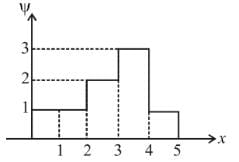What is the probability that the particle would be found between x = 2 and x = 4 ?

Detailed Solution for Postulates Of Quantum Mechanics MCQ Level - 2 - Question 4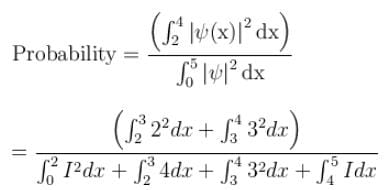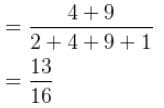Postulates Of Quantum Mechanics MCQ Level - 2 - Question 5

The probability current density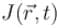is given as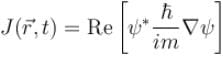Which of the following is true?

Detailed Solution for Postulates Of Quantum Mechanics MCQ Level - 2 - Question 5

From the continuity equation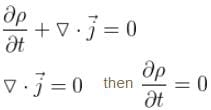i.e. p is constant in time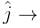Probability current density
The correct answer is: If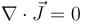then probability density is constant in time.

Postulates Of Quantum Mechanics MCQ Level - 2 - Question 6

Smallest possible uncertainty in position of the electron moving with velocity 3 × 107 m/s. Given, h = 6.63 × 10–34 Js, m0 = 9.1 × 10–31 kg.

Detailed Solution for Postulates Of Quantum Mechanics MCQ Level - 2 - Question 6

Given, v = 3 × 107 m/s
Let    Δxmin be the minimum uncertainty in position of the electron and Δp the maximum uncertainty in the momentum of the electron.

Thus, we have,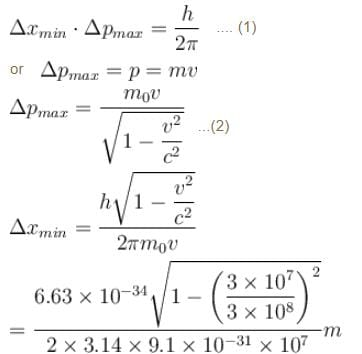= 0.03867 × 0.9949 × 10–10 m
= 3.8 × 10–12 m
The correct answer is: 3.8 × 10–12 m

Postulates Of Quantum Mechanics MCQ Level - 2 - Question 7

Choose the correct statement

Detailed Solution for Postulates Of Quantum Mechanics MCQ Level - 2 - Question 7

The correct answer is: All the energy eigenvalues are always real.

Postulates Of Quantum Mechanics MCQ Level - 2 - Question 8

The wave function of the particle lies in which region?

Detailed Solution for Postulates Of Quantum Mechanics MCQ Level - 2 - Question 8

The particle cannot exist outside the box, as it cannot have infinite amount of energy. Thus, it’s wave function is between 0 and L, where L is the length of the side of the box.

Postulates Of Quantum Mechanics MCQ Level - 2 - Question 9

A linear harmonic oscillator of mass m oscillates with a frequency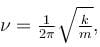where k is its force constant. What is the minimum energy of the oscillator.

Detailed Solution for Postulates Of Quantum Mechanics MCQ Level - 2 - Question 9

The energy of the linear harmonic oscillator is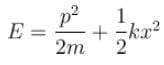This is a constant of motion. We can represent the constant value of E by means of averages of the kinetic and potential over a cycle of motion by writing.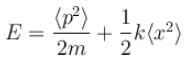The average value of x and p should vanish for an oscillating particle. So, we can identify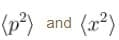with the square of the corresponding uncertainties.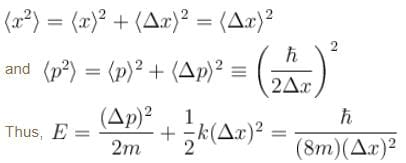Since from the uncertainty principle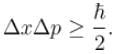To determine the minimum energy of the oscillator, we put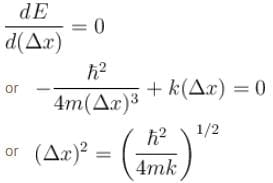The minimum energy is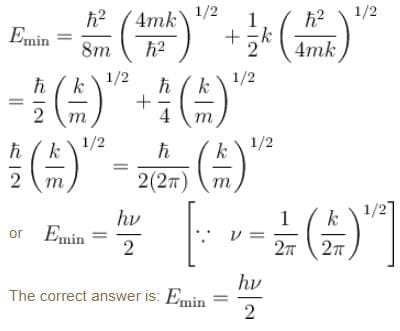Postulates Of Quantum Mechanics MCQ Level - 2 - Question 10

Choose the correct statement for a free particle with  ψ(x) = Aeikx

Detailed Solution for Postulates Of Quantum Mechanics MCQ Level - 2 - Question 10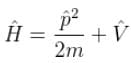V = 0 for a free particle
∴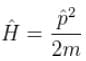Schrödinger equation.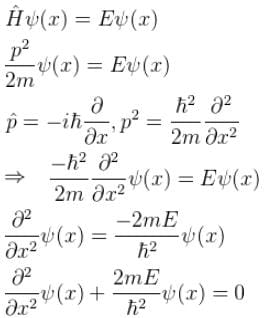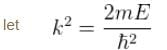∴  Aeikx and Be–ikx     (General solution)
∴  are solution to the free particle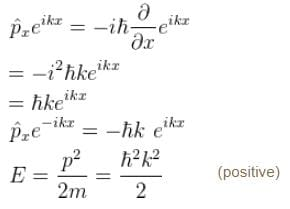The correct answer is: Energy eigenvalue is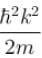and momentum is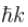Information about Postulates Of Quantum Mechanics MCQ Level - 2 Page
In this test you can find the Exam questions for Postulates Of Quantum Mechanics MCQ Level - 2 solved & explained in the simplest way possible. Besides giving Questions and answers for Postulates Of Quantum Mechanics MCQ Level - 2, EduRev gives you an ample number of Online tests for practice(Scan QR code)